Next: Time-dependent density functional theory Up: Continuity equation in the Previous: Continuity equation in the

## Time evolution of a spin-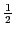particle

We begin by recalling the well-known  CE for a single particle. The time evolution of a non-relativistic spin-particle moving in a local potential is given by the Schrödinger equation,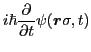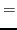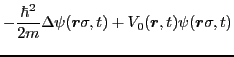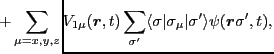(1)

where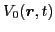and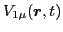are scalar and vector real time-dependent potentials, respectively, and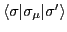are the standard Pauli matrices. By multiplying Eq. (1) with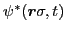, summing up over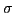, and taking the imaginary part, we obtain the standard CE for the probability density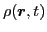in terms of the current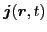,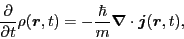(2)

where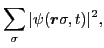(3)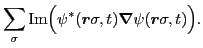(4)

We see that the hermiticity of the local potential guarantees that the potential energy does not contribute to the CE of Eq. (2).

Similarly, by multiplying Eq. (1) with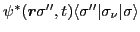, summing up over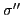and, and taking the imaginary part, we obtain the CE for the spin density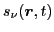in terms of the spin current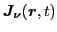,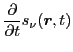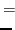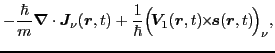(5)

where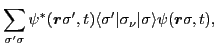(6)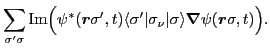(7)

We see that the spin CE does depend on the vector potential, and the second term in Eq. (5) is responsible, e.g., for the spin precession in magnetic field.

It is interesting to note that when potential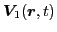is parallel to the spin density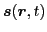(non-linear Schrödinger equation), all components of the spin density fulfill the CEs. In fact, this is exactly the case for the TDHF equation induced by a zero-range two-body interaction, see below. Another interesting case corresponds to the vector potential aligned along a fixed direction in space, say, along the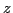axis, that is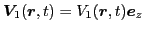. In this case, the time evolutions of the spin-up and spin-down components decouple from one another, that is,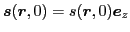implies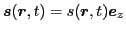, and the spin-up and spin-down components individually obey the corresponding CEs.

We also note here that for a nonlocal potential-energy term,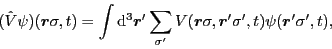(8)

the time evolution does not, in general, lead to a CE.Next: Time-dependent density functional theory Up: Continuity equation in the Previous: Continuity equation in the
Jacek Dobaczewski 2011-11-11